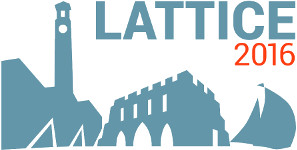#The 34th International Symposium on Lattice Field Theory (Lattice 2016)

Jul 24 – 30, 2016
Highfield Campus, University of Southampton
Europe/London timezone

## Prediction of positive parity Bs mesons and search for the X(5568)

Jul 26, 2016, 8:00 PM
1h
Highfield Campus, University of Southampton

#### Highfield Campus, University of Southampton

Highfield Campus, Southampton SO17 1BJ, UK

### Speaker

Dr Daniel Mohler (Helmholtz-Institut Mainz)

### Description

We use a combination of quark-antiquark and $B^{(*)}K$ interpolating fields to predict the mass of two QCD bound states below the $B^*K$ threshold in the quantum channels $J^P=0^+$ and $1^+$. The mesons correspond to the b-quark cousins of the $D_{s0}^*(2317)$ and $D_{s1}(2460)$ and have not yet been observed in experiment, even though they are expected to be found by LHCb. In addition to these predictions, we obtain excellent agreement of the remaining p-wave energy levels with the known $B_{s1}(5830)$ and $B_{s2}^*(5840)$ mesons. The results from our first principles calculation are compared to previous model-based estimates. More recently the D0 collaboration claimed the existence of an exotic resonance $X(5568)$ with exotic flavor content $\bar{b}s\bar{d}u$. If such a state with $J^P=0^+$ exists, only the decay into $B_s\pi$ is open which makes a lattice search for this state much cleaner and simpler than for other exotic candidates involving heavy quarks. We conclude, however, that we do not find such a candidate in agreement with a recent LHCb result.

### Primary author

Dr Daniel Mohler (Helmholtz-Institut Mainz)

### Co-authors

Prof. C. B. Lang (Karl-Franzens-Universitaet Graz) Sasa Prelovsek (University of Ljubljana)

 Poster